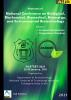# Computation of gamma ray mass attenuation coefficients and exposure build-up factor of hybrid biomaterials

## Authors

Sandeep Gupta
Department of Physics, Punjabi University College, Dhilwan (Barnala) Punjab

### Synopsis

The energy parameters of partial interaction cross section and total mass attenuation coefficients (µtot.) of hybrid bio-materials such as bioglass solution [HB1] and PVA-Bioactive glass hybrid foams [HB2] was calculated by using the ‘WinXcom’ computer software over the gamma energy range 1 KeV to 100 GeV. For calculating gamma-ray energy exposure buildup factor (EBF) of chosen samples, Geometric progression (GP) method was used for the energy range 0.015–15 MeV and penetration depths upto 40mfp. With the help of graphs and equations describing the Z (atomic no.) dependency, the density of various chosen samples can be ascertained.  It has been concluded that the values of exposure buildup factor are very large in the medium incident photon energy range, where Compton scattering process is dominant and the values of EBF are less in both the lower incident photon energy range where photoelectric absorption process is dominant and the higher incident photon energy range where Pair production process is dominant.Published
March 16, 2021